xLaw of large numbersOverview

In probability theory
Probability theory
Probability theory is the branch of mathematics concerned with analysis of random phenomena. The central objects of probability theory are random variables, stochastic processes, and events: mathematical abstractions of non-deterministic events or measured quantities that may either be single...

, the law of large numbers (LLN) is a theorem
Theorem
In mathematics, a theorem is a statement that has been proven on the basis of previously established statements, such as other theorems, and previously accepted statements, such as axioms...

that describes the result of performing the same experiment a large number of times. According to the law, the average
Average
In mathematics, an average, or central tendency of a data set is a measure of the "middle" value of the data set. Average is one form of central tendency. Not all central tendencies should be considered definitions of average....

of the results obtained from a large number of trials should be close to the expected value
Expected value
In probability theory, the expected value of a random variable is the weighted average of all possible values that this random variable can take on...

, and will tend to become closer as more trials are performed.

For example, a single roll of a six-sided produces one of the numbers 1, 2, 3, 4, 5, 6, each with equal probability
Probability
Probability is ordinarily used to describe an attitude of mind towards some proposition of whose truth we arenot certain. The proposition of interest is usually of the form "Will a specific event occur?" The attitude of mind is of the form "How certain are we that the event will occur?" The...

.Unanswered QuestionsDoes the lln support that 15% of the total population will vote in our next presidrntial election???Encyclopedia
In probability theory
Probability theory
Probability theory is the branch of mathematics concerned with analysis of random phenomena. The central objects of probability theory are random variables, stochastic processes, and events: mathematical abstractions of non-deterministic events or measured quantities that may either be single...

, the law of large numbers (LLN) is a theorem
Theorem
In mathematics, a theorem is a statement that has been proven on the basis of previously established statements, such as other theorems, and previously accepted statements, such as axioms...

that describes the result of performing the same experiment a large number of times. According to the law, the average
Average
In mathematics, an average, or central tendency of a data set is a measure of the "middle" value of the data set. Average is one form of central tendency. Not all central tendencies should be considered definitions of average....

of the results obtained from a large number of trials should be close to the expected value
Expected value
In probability theory, the expected value of a random variable is the weighted average of all possible values that this random variable can take on...

, and will tend to become closer as more trials are performed.

For example, a single roll of a six-sided produces one of the numbers 1, 2, 3, 4, 5, 6, each with equal probability
Probability
Probability is ordinarily used to describe an attitude of mind towards some proposition of whose truth we arenot certain. The proposition of interest is usually of the form "Will a specific event occur?" The attitude of mind is of the form "How certain are we that the event will occur?" The...

. Therefore, the expected value of a single dice roll is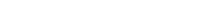According to the law of large numbers, if a large number of six-sided dice are rolled, the average of their values (sometimes called the sample mean) is likely to be close to 3.5, with the accuracy increasing as more dice are rolled. This assumes that all possible die roll outcomes are known and that Black Swan events
Black swan theory
The black swan theory or theory of black swan events is a metaphor that encapsulates the concept that The event is a surprise and has a major impact...

such as a die landing on edge or being struck by lightning mid-roll are not possible or ignored if they do occur.

It follows from the law of large numbers that the empirical probability
Empirical probability
Empirical probability, also known as relative frequency, or experimental probability, is the ratio of the number of "favorable" outcomes to the total number of trials, not in a sample space but in an actual sequence of experiments...

of success in a series of Bernoulli trials will converge to the theoretical probability. For a Bernoulli random variable, the expected value is the theoretical probability of success, and the average of n such variables (assuming they are independent and identically distributed (i.i.d.)
Independent and identically distributed random variables
In probability theory and statistics, a sequence or other collection of random variables is independent and identically distributed if each random variable has the same probability distribution as the others and all are mutually independent....

) is precisely the relative frequency.

For example, a fair coin
Fair coin
In probability theory and statistics, a sequence of independent Bernoulli trials with probability 1/2 of success on each trial is metaphorically called a fair coin. One for which the probability is not 1/2 is called a biased or unfair coin...

toss is a Bernoulli trial
Bernoulli trial
In the theory of probability and statistics, a Bernoulli trial is an experiment whose outcome is random and can be either of two possible outcomes, "success" and "failure"....

. When a fair coin is flipped once, the theoretical probability that the outcome will be heads is equal to 1/2. Therefore, according to the law of large numbers, the proportion of heads in a "large" number of coin flips "should be" roughly 1/2. In particular, the proportion of heads after n flips will almost surely
Almost surely
In probability theory, one says that an event happens almost surely if it happens with probability one. The concept is analogous to the concept of "almost everywhere" in measure theory...

converge
Limit of a sequence
The limit of a sequence is, intuitively, the unique number or point L such that the terms of the sequence become arbitrarily close to L for "large" values of n...

to 1/2 as n approaches infinity.

Though the proportion of heads (and tails) approaches 1/2, almost surely the absolute (nominal) difference
Absolute difference
The absolute difference of two real numbers x, y is given by |x − y|, the absolute value of their difference. It describes the distance on the real line between the points corresponding to x and y...

in the number of heads and tails will become large as the number of flips becomes large. That is, the probability that the absolute difference is a small number, approaches zero as the number of flips becomes large. Also, almost surely the ratio of the absolute difference to the number of flips will approach zero. Intuitively, expected absolute difference grows, but at a slower rate than the number of flips, as the number of flips grows.

The LLN is important because it "guarantees" stable long-term results for random events. For example, while a casino may lose money in a single spin of the roulette
Roulette
Roulette is a casino game named after a French diminutive for little wheel. In the game, players may choose to place bets on either a single number or a range of numbers, the colors red or black, or whether the number is odd or even....

wheel, its earnings will tend towards a predictable percentage over a large number of spins. Any winning streak by a player will eventually be overcome by the parameters of the game. It is important to remember that the LLN only applies (as the name indicates) when a large number of observations are considered. There is no principle that a small number of observations will converge to the expected value or that a streak of one value will immediately be "balanced" by the others. See the Gambler's fallacy
Gambler's fallacy
The Gambler's fallacy, also known as the Monte Carlo fallacy , and also referred to as the fallacy of the maturity of chances, is the belief that if deviations from expected behaviour are observed in repeated independent trials of some random process, future deviations in the opposite direction are...

.

## History

The Italian mathematician Gerolamo Cardano
Gerolamo Cardano
Gerolamo Cardano was an Italian Renaissance mathematician, physician, astrologer and gambler...

(1501–1576) stated without proof that the accuracies of empirical statistics tend to improve with the number of trials. This was then formalized as a law of large numbers. A special form of the LLN (for a binary random variable) was first proved by Jacob Bernoulli. It took him over 20 years to develop a sufficiently rigorous mathematical proof which was published in his Ars Conjectandi
Ars Conjectandi
Ars Conjectandi is a combinatorial mathematical paper written by Jakob Bernoulli and published in 1713, eight years after his death, by his nephew, Niklaus Bernoulli. The seminal work consolidated, most notably among other combinatorial topics, probability theory: indeed, it is widely regarded as...

(The Art of Conjecturing) in 1713. He named this his "Golden Theorem" but it became generally known as "Bernoulli's Theorem". This should not be confused with the principle in physics with the same name
Bernoulli's principle
In fluid dynamics, Bernoulli's principle states that for an inviscid flow, an increase in the speed of the fluid occurs simultaneously with a decrease in pressure or a decrease in the fluid's potential energy...

, named after Jacob Bernoulli's nephew Daniel Bernoulli
Daniel Bernoulli
Daniel Bernoulli was a Dutch-Swiss mathematician and was one of the many prominent mathematicians in the Bernoulli family. He is particularly remembered for his applications of mathematics to mechanics, especially fluid mechanics, and for his pioneering work in probability and statistics...

. In 1837, S.D. Poisson
Siméon Denis Poisson
Siméon Denis Poisson , was a French mathematician, geometer, and physicist. He however, was the final leading opponent of the wave theory of light as a member of the elite l'Académie française, but was proven wrong by Augustin-Jean Fresnel.-Biography:...

further described it under the name "la loi des grands nombres" ("The law of large numbers"). Thereafter, it was known under both names, but the "Law of large numbers" is most frequently used.

After Bernoulli and Poisson published their efforts, other mathematicians also contributed to refinement of the law, including Chebyshev
Pafnuty Chebyshev
Pafnuty Lvovich Chebyshev was a Russian mathematician. His name can be alternatively transliterated as Chebychev, Chebysheff, Chebyshov, Tschebyshev, Tchebycheff, or Tschebyscheff .-Early years:One of nine children, Chebyshev was born in the village of Okatovo in the district of Borovsk,...

, Markov
Andrey Markov
Andrey Andreyevich Markov was a Russian mathematician. He is best known for his work on theory of stochastic processes...

, Borel
Émile Borel
Félix Édouard Justin Émile Borel was a French mathematician and politician.Borel was born in Saint-Affrique, Aveyron. Along with René-Louis Baire and Henri Lebesgue, he was among the pioneers of measure theory and its application to probability theory. The concept of a Borel set is named in his...

, Cantelli
Francesco Paolo Cantelli
Francesco Paolo Cantelli was an Italian mathematician. He was the founder of the Istituto Italiano degli Attuari for the applications of mathematics and probability to economics....

and Kolmogorov
Andrey Kolmogorov
Andrey Nikolaevich Kolmogorov was a Soviet mathematician, preeminent in the 20th century, who advanced various scientific fields, among them probability theory, topology, intuitionistic logic, turbulence, classical mechanics and computational complexity.-Early life:Kolmogorov was born at Tambov...

and Khinchin (who finally provided a complete proof of the LLN for the arbitrary random variables). These further studies have given rise to two prominent forms of the LLN. One is called the "weak" law and the other the "strong" law. These forms do not describe different laws but instead refer to different ways of describing the mode of convergence
Limit of a sequence
The limit of a sequence is, intuitively, the unique number or point L such that the terms of the sequence become arbitrarily close to L for "large" values of n...

of the cumulative sample means to the expected value, and the strong form implies the weak.

## Forms

Two different versions of the Law of Large Numbers are described below; they are called the Strong Law of Large Numbers, and the Weak Law of Large Numbers.
Both versions of the law state that – with virtual certainty – the sample average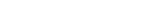converges to the expected value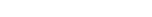where X1, X2, ... is an infinite sequence of i.i.d. integrable random variables with expected value E(X1) = E(X2) = ...= µ. Integrability means that E(|X|1) = E(|X|2) = ... < ∞.

An assumption of finite variance
Variance
In probability theory and statistics, the variance is a measure of how far a set of numbers is spread out. It is one of several descriptors of a probability distribution, describing how far the numbers lie from the mean . In particular, the variance is one of the moments of a distribution...

Var(X1) = Var(X2) = ... = σ2 < ∞ is not necessary. Large or infinite variance will make the convergence slower, but the LLN holds anyway. This assumption is often used because it makes the proofs easier and shorter.

The difference between the strong and the weak version is concerned with the mode of convergence being asserted. For interpretation of these modes, see Convergence of random variables
Convergence of random variables
In probability theory, there exist several different notions of convergence of random variables. The convergence of sequences of random variables to some limit random variable is an important concept in probability theory, and its applications to statistics and stochastic processes...

.

### Weak law

The weak law of large numbers states that the sample average converges in probability  towards the expected value[proof]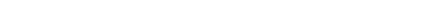That is to say that for any positive number ε,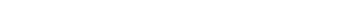Interpreting this result, the weak law essentially states that for any nonzero margin specified, no matter how small, with a sufficiently large sample there will be a very high probability that the average of the observations will be close to the expected value, that is, within the margin.

Convergence in probability is also called weak convergence of random variables. This version is called the weak law because random variables may converge weakly (in probability) as above without converging strongly (almost surely) as below.

### Strong law

The strong law of large numbers states that the sample average converges almost surely to the expected value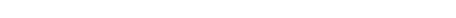That is,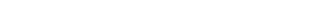The proof is more complex than that of the weak law. This law justifies the intuitive interpretation of the expected value of a random variable as the "long-term average when sampling repeatedly".

Almost sure convergence is also called strong convergence of random variables. This version is called the strong law because random variables which converge strongly (almost surely) are guaranteed to converge weakly (in probability). The strong law implies the weak law.

The strong law of large numbers can itself be seen as a special case of the pointwise ergodic theorem.

Moreover, if the summands are independent but not identically distributed, then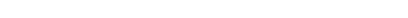provided that each Xk has a finite second moment and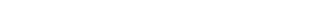This statement is known as Kolmogorov's strong law, see e.g. .

### Differences between the weak law and the strong law

The weak law states that for a specified large n, the average $\overline{X}_n$ is likely to be near μ. Thus, it leaves open the possibility that $|\overline{X}_n -\mu| > \varepsilon$ happens an infinite number of times, although at infrequent intervals.

The strong law shows that this almost surely
Almost surely
In probability theory, one says that an event happens almost surely if it happens with probability one. The concept is analogous to the concept of "almost everywhere" in measure theory...

will not occur. In particular, it implies that with probability 1, we have that for any the inequality $|\overline{X}_n -\mu| < \varepsilon$ holds for all large enough n.

### Uniform law of large numbers

Suppose f(x,θ) is some function
Function (mathematics)
In mathematics, a function associates one quantity, the argument of the function, also known as the input, with another quantity, the value of the function, also known as the output. A function assigns exactly one output to each input. The argument and the value may be real numbers, but they can...

defined for θ ∈ Θ, and continuous in θ. Then for any fixed θ, the sequence {f(X1,θ), f(X2,θ), …} will be a sequence of independent and identically distributed random variables, such that the sample mean of this sequence converges in probability to E[f(X,θ)]. This is the pointwise (in θ) convergence.

The uniform law of large numbers states the conditions under which the convergence happens uniformly in θ. If

1. Θ is compact,
2. f(x,θ) is continuous at each θ ∈ Θ for almost all
Almost everywhere
In measure theory , a property holds almost everywhere if the set of elements for which the property does not hold is a null set, that is, a set of measure zero . In cases where the measure is not complete, it is sufficient that the set is contained within a set of measure zero...

x’s,
3. there exists a dominating function d(x) such that E[d(X)] < ∞, and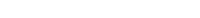Then E[f(X,θ)] is continuous in θ, and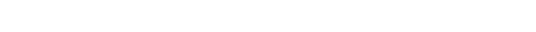### Borel's law of large numbers

Borel's law of large numbers, named after Émile Borel
Émile Borel
Félix Édouard Justin Émile Borel was a French mathematician and politician.Borel was born in Saint-Affrique, Aveyron. Along with René-Louis Baire and Henri Lebesgue, he was among the pioneers of measure theory and its application to probability theory. The concept of a Borel set is named in his...

, states that if an experiment is repeated a large number of times, independently under identical conditions, then the proportion of times that any specified event occurs approximately equals the probability of the event's occurrence on any particular trial; the larger the number of repetitions, the better the approximation tends to be. More precisely, if E denotes the event in question, p its probability of occurrence, and Nn(E) the number of times E occurs in the first n trials, then with probability one,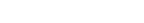This theorem makes rigorous the intuitive notion of probability as the long-run relative frequency of an event's occurrence. It is a special case of any of several more general laws of large numbers in probability theory.

## Proof

Given X1, X2, ... an infinite sequence of i.i.d. random variables with finite expected value E(X1) = E(X2) = ... = µ < ∞, we are interested in the convergence of the sample average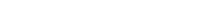The weak law of large numbers states:

Theorem: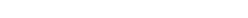### Proof using Chebyshev's inequality

This proof uses the assumption of finite variance
Variance
In probability theory and statistics, the variance is a measure of how far a set of numbers is spread out. It is one of several descriptors of a probability distribution, describing how far the numbers lie from the mean . In particular, the variance is one of the moments of a distribution...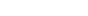(for all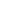). The independence of the random variables implies no correlation between them, and we have that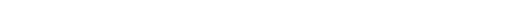The common mean μ of the sequence is the mean of the sample average: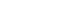Using Chebyshev's inequality
Chebyshev's inequality
In probability theory, Chebyshev’s inequality guarantees that in any data sample or probability distribution,"nearly all" values are close to the mean — the precise statement being that no more than 1/k2 of the distribution’s values can be more than k standard deviations away from the mean...

on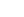results in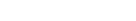This may be used to obtain the following: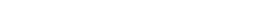As n approaches infinity, the expression approaches 1. And by definition of convergence in probability (see Convergence of random variables
Convergence of random variables
In probability theory, there exist several different notions of convergence of random variables. The convergence of sequences of random variables to some limit random variable is an important concept in probability theory, and its applications to statistics and stochastic processes...

), we have obtained### Proof using convergence of characteristic functions

By Taylor's theorem
Taylor's theorem
In calculus, Taylor's theorem gives an approximation of a k times differentiable function around a given point by a k-th order Taylor-polynomial. For analytic functions the Taylor polynomials at a given point are finite order truncations of its Taylor's series, which completely determines the...

for complex functions, the characteristic function
Characteristic function (probability theory)
In probability theory and statistics, the characteristic function of any random variable completely defines its probability distribution. Thus it provides the basis of an alternative route to analytical results compared with working directly with probability density functions or cumulative...

of any random variable, X, with finite mean μ, can be written as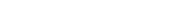All X1, X2, ... have the same characteristic function, so we will simply denote this φX.

Among the basic properties of characteristic functions there are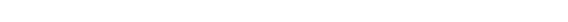These rules can be used to calculate the characteristic function of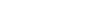in terms of φX: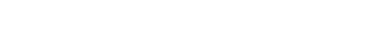The limit  eitμ  is the characteristic function of the constant random variable μ, and hence by the Lévy continuity theorem
Lévy continuity theorem
In probability theory, the Lévy’s continuity theorem, named after the French mathematician Paul Lévy, connects convergence in distribution of the sequence of random variables with pointwise convergence of their characteristic functions...

,converges in distribution to μ: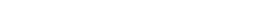μ is a constant, which implies that convergence in distribution to μ and convergence in probability to μ are equivalent. (See Convergence of random variables
Convergence of random variables
In probability theory, there exist several different notions of convergence of random variables. The convergence of sequences of random variables to some limit random variable is an important concept in probability theory, and its applications to statistics and stochastic processes...

) This implies thatThis proof states, in fact, that the sample mean converges in probability to the derivative of the characteristic function at the origin, as long as this exists.

## See also

• Central limit theorem
Central limit theorem
In probability theory, the central limit theorem states conditions under which the mean of a sufficiently large number of independent random variables, each with finite mean and variance, will be approximately normally distributed. The central limit theorem has a number of variants. In its common...

• Law of the iterated logarithm
Law of the iterated logarithm
In probability theory, the law of the iterated logarithm describes the magnitude of the fluctuations of a random walk. The original statement of the law of the iterated logarithm is due to A. Y. Khinchin . Another statement was given by A.N...

• Infinite monkey theorem
Infinite monkey theorem
The infinite monkey theorem states that a monkey hitting keys at random on a typewriter keyboard for an infinite amount of time will almost surely type a given text, such as the complete works of William Shakespeare....

• Law of averages
Law of averages
The law of averages is a lay term used to express a belief that outcomes of a random event will "even out" within a small sample.As invoked in everyday life, the "law" usually reflects bad statistics or wishful thinking rather than any mathematical principle...

## External links

• Animations for the Law of Large Numbers by Yihui Xie using the R
R (programming language)
R is a programming language and software environment for statistical computing and graphics. The R language is widely used among statisticians for developing statistical software, and R is widely used for statistical software development and data analysis....

package animation
The source of this article is wikipedia, the free encyclopedia.  The text of this article is licensed under the GFDL.